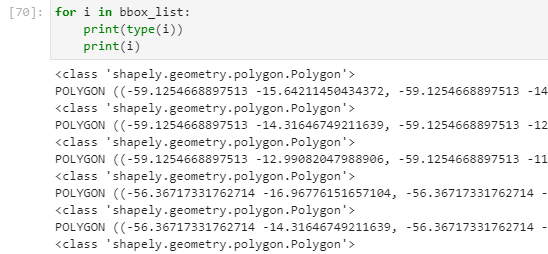# Shapely bbox geometry to sentinelhub bbox

I have list of polygons that are shapely type. These polygons are different boudning boxes that I have slected for certain process:I want to have these polygons as bbox type. For that I have tried to use the get_bbox_from_shape function, but that doesn’t work as I understand it needs to have sentinelhub geometry:

``````def get_bbox_from_shape(shape,resolution):
minx, miny, maxx, maxy = shape.geometry.total_bounds
bbox_coords_wgs84=[minx, miny, maxx, maxy]
bbox = BBox(bbox=bbox_coords_wgs84, crs=CRS.WGS84)
bbox_size = bbox_to_dimensions(bbox, resolution=resolution)
return bbox_size,bbox,bbox_coords_wgs84

get_bbox_from_shape(bbox_list,10)
``````

AttributeError Traceback (most recent call last)
in
----> 1 get_bbox_from_shape(bbox_list,10)

in get_bbox_from_shape(shape, resolution)
1 def get_bbox_from_shape(shape,resolution):
----> 2 minx, miny, maxx, maxy = shape.geometry.total_bounds
3 bbox_coords_wgs84=[minx, miny, maxx, maxy]
4 bbox = BBox(bbox=bbox_coords_wgs84, crs=CRS.WGS84)
5 bbox_size = bbox_to_dimensions(bbox, resolution=resolution)

AttributeError: ‘Polygon’ object has no attribute ‘geometry’

So I have tried to convert my polygon into sentinelhub geoemtry in order to be able to get sentinelhub bbox type:

``````from shapely.geometry import shape, Polygon, MultiPolygon, MultiLineString
from sentinelhub import BBox, read_data, CRS, DataCollection,bbox_to_dimensions,geometry

for i in bbox_list:
test=sentinelhub.geoemtry.Geoemtry(i,CRS.WGS84)
``````

However, that return error:
NameError: name ‘sentinelhub’ is not defined

I’m not sure why I recieve this error as I have sentinelhub imported.
How can I convert my list f shapely bbox into a bbox that I can work with in sentinelhub?

maybe there is better/easier way to convert it?

**My end goal : to convert shapely bbox geometries into bbox I can work with with sentinelhub (class ‘sentinelhub.geometry.BBox’) **

There is a small error in your `get_bbox_from_shape` function: `shape` is already a shapely geometry, and as such, has no attribute `geometry` (this is what the first error says: AttributeError: ‘Polygon’ object has no attribute ‘geometry’)

Changing the line

``````minx, miny, maxx, maxy = shape.geometry.total_bounds
``````

to

``````minx, miny, maxx, maxy = shape.bounds
``````

works.

Beware that your code works correctly only for geometries that are in WGS84 coordinate reference system.

Your second approach is also possible, and has an error due to imports… If you do

``````from sentinelhub import BBox, CRS, Geometry
``````

you can create bounding boxes from your geometries as

``````bbox = Geometry(shape, CRS.WGS84).bbox
``````

(`shape` would be `i` in your for loop).

1 Like